# TECH SPOT: SAMPLE CRE QUESTIONS (Part 20)1. Which of the following is most valuable in Human engineering?:
A.FMECA
B. Digital computer
C. Monte Carlo Analysis
D. Full scale model

2. Hazard probability level “A” assigned to a hazard normally means: The Hazard …..
A. Is possible
B. Is unlikely to occur
C. Is very likely to occur
D. Will occur sometimes

3.The purpose of a burn-in operation on parts:
A Make sure they can operate at elevated temperature.
B. Make sure they can meet safety regulations
C. Remove weak members from the population.
D. Determine their thermal resistance

4. Five motors are run to failure. Failure times are (in hours): 632,3450,816,928,150. Assuming an exponential distribution, what are the 90% confidence bounds for the MTTF?
A. 1051, 1942
B. 847, 2650
C. 347, 3747
D. 653, 3034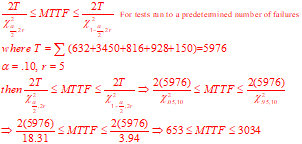5. The reliability of a machine is given by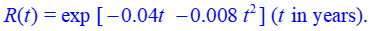What is the failure rate?
A.0.04-0.008t
B. .04+.016t
C. .0016+. 008t2
D. -.04+.064t

𝑅(𝑡)=𝑒^(−0.04𝑡−0.008𝑡^2 )
𝑓(𝑡)=−𝑑𝑅(𝑡)/𝑑𝑡=(0.04+0.016𝑡) 𝑒^(−0.04𝑡−0.008𝑡^2 );𝜆(𝑡)=𝑓(𝑡)/𝑅(𝑡) =0.04+0.016𝑡

6. There is a contractual requirement to demonstrate with 90% confidence that a vehicle can achieve a 100-km mission with a reliability of 99%. The acceptance test is performed by running 10 vehicles over a 50,000- km test track.
What is the contractual MTTF?
A. 9950km
B. 4975km
C. 19900km
D. 7500km

𝑅(𝑇)=0.99=𝑒^(−𝜆𝑥)=𝑒^(−𝑥/𝑀𝑇𝑇𝐹)→−𝜆/𝑀𝑇𝑇𝐹=ln⁡0.9 → 𝑀𝑇𝑇𝐹=−100/ln⁡0.99 =9,949.9 𝑘𝑚

7. Suppose the CDF for time to failure is given by: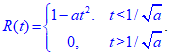Determine the PDF f(t),
A. f(t)=at
B. f(t)=2-at
C. f(t)=2at
D. f(t)=1/Sqrt(at)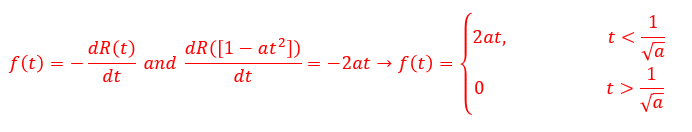8. A manufacturer determines that the average television set is used 1.8 hr/day. A one-year warranty is offered on the picture tube having a MTTF of 2000 hr. If the distribution is exponential, what fraction of the tubes will fail during the warranty period?
A. 0.28
B. 0.17
C. 0.34
D. 0.48

Hours of use in one year=365×1.8=657 h ⇒ 𝐹=1−𝑒^(−𝜆𝑡)=1−𝑒^(−657/2,000)=0.28

9. Assume that the occurrence of earthquakes strong enough to be damaging to a particular structure is governed by the Poisson distribution. If the mean time between such earth quakes is twice the design life of the structure, what is the probability that the structure will be damaged during its design life?
A. .90
B. .73
C. .61
D. .50

𝑅𝑒𝑙𝑖𝑎𝑏𝑖𝑙𝑖𝑡𝑦 (𝐷𝑒𝑠𝑖𝑔𝑛 𝐿𝑖𝑓𝑒)=𝑒^(−𝜆𝑇)=𝑒^(−1/𝑇 𝑇)

“At twice the earthquake interval Reliability ” (“Design Life” )=𝑒^(−𝜆_2 𝑇)=𝑒^(−1/2𝑇 𝑇)=0.6065

10. Thermocouples of a particular design have a failure rate of λ = 0.008/ hr. How many thermocouples must be placed in active parallel if the system is to run for 100 hrs with a system failure probability of no more than 0.05? Assume that all failures are independent.
A. 4
B. 5
C. 6
D. 7

Component Reliability, 𝑅=𝑒^(−𝜆𝑡)=𝑒^(−0.008×100)=0.4493

𝐹≤0.05→(1−𝑅)^𝑁≤0.05, 𝑁 ln⁡(1−𝑅)≤ln⁡0.05→𝑁≥5.02 ~ 6 units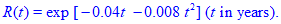## More To ExploreSample Questions

### TECH SPOT: SAMPLE CRE QUESTIONS (Part 21)

1. A system made up of 5 series components has a design reliability of .96. Subsequently, 4 of the components have reliabilities apportioned to them of 0.992, 0.991, 0.994, and 0.992. What should the reliability apportionment for the 5th subsystem be?A. 0.970 B. 0.969 C. 0.990 D. 0.992   2. Which process has as itsSample Questions

### TECH SPOT: SAMPLE CRE QUESTIONS (Part 20)

1. Which of the following is most valuable in Human engineering?: A.FMECA B. Digital computer C. Monte Carlo Analysis D. Full scale model 2. Hazard probability level “A” assigned to a hazard normally means: The Hazard ….. A. Is possible B. Is unlikely to occur C. Is very likely to occur D. Will occur sometimes

Scroll to Top